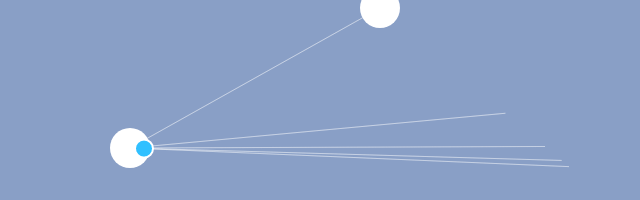# Simplest possible predictive aimingThis is a blog post about aiming ahead to hit moving targets! It has interactive elements.

## Explanation

Let's suppose your ultimate nemesis stole your lunch and is running away with it.

As they are about to leave your field of view, you recall that you have a water balloon.

It's a "now or never" kind of moment. You have to act.

Let's start with figuring out what you would do in this situation yourself:

There would usually be an interactive demo here,
but your browser doesn't support HTML5but you have JavaScript disabled 😒
(click to interact)

With a bit of logical thinking and a few attempts, justice can be served.

Now, let's see how could we make the computer do the same.

As you might guess, simply aiming at the target's current position isn't going to work unless the projectile is very fast, the target is very slow, or you are really close to the target:

(click to interact)(mouseover/click to play GIF)

The next step would be to guess where the target should be by the time the projectile reaches it:

time to reach = (distance to target) / (projectile's speed)
direction = direction to ((target's starting position) + (target's speed) * (time to reach))

(click to interact)(mouseover/click to play GIF)

As can be seen, that didn't quite work. And why? We calculated where the target should be, but the time to reach that new position is different, and thus we might miss.

There are a few ways out of this situation:
A "true" solution would be to switch to a slightly better interception or steering behaviour formula. A simpler (and slightly more universal) solution is to simply repeat the calculation for the updated position - each iteration increases the precision, and, after doing so 3-4 times,

temp position = target position
repeat 4 times:

• time to reach = (distance to temp position) / (projectile's speed)
• temp position = ((target's starting position) + (target's speed) * (time to reach))

direction = direction to (temp position)

we get the right point to hit the target.

(click to interact)(mouseover/click to play GIF)

This can be also adapted for other kinds of motion - for example, if the target was to move in an arc with initial velocity and gravity, the formula would be updated to:

temp position = target position
repeat 4 times:

• t[ime to reach] = (distance to temp position) / (projectile's speed)
• temp position X = ((target's starting position X) + (target's initial X speed) * t)
• temp position Y = ((target's starting position Y) + (target's initial Y speed) * t + gravity / 2 * t²)

direction = direction to (temp position)

(click to interact)(mouseover/click to play GIF)

Or, if you had the target move along a Bezier curve, you could sample new coordinates along the path and have that work too:(mouseover/click to play GIF)

As a note here, for paths with extreme curvature you may want to update the temp position to be halfway between old and new estimates (as typical binary search would) to avoid some oddities and save on iterations required.

## Code examples

The following are for GameMaker.

For using outside of GameMaker, point_distance(x1, y1, x2, y2) is defined as

```var dx = x2 - x1, dy = y2 - y1;
return sqrt(dx * dx + dy * dy); // square root
```

and point_direction(x1, y1, x2, y2) is defined as

```var dx = x2 - x1, dy = y2 - y1;
return arctan2(dy, dx); // https://en.wikipedia.org/wiki/Atan2
```

Picking direction for a linear path:

```/// @param _bullet_x
/// @param _bullet_y
/// @param _bullet_speed
/// @param _target_x
/// @param _target_y
/// @param _target_xsp
/// @param _target_ysp
var _bullet_x = argument0, _bullet_y = argument1, _bullet_speed = argument2, _target_x = argument3, _target_y = argument4, _target_xsp = argument5, _target_ysp = argument6;
var _est_x = _target_x;
var _est_y = _target_y;
var _est_dist, _est_time;
repeat (16) { // <- max iteration count
_est_dist = point_distance(_bullet_x, _bullet_y, _est_x, _est_y);
_est_time = _est_dist / _bullet_speed;
var _old_x = _est_x;
var _old_y = _est_y;
_est_x = _target_x + _target_xsp * _est_time;
_est_y = _target_y + _target_ysp * _est_time;
// early exit if we're "close enough":
if (point_distance(_old_x, _old_y, _est_x, _est_y) < 1) break;
}
// the following can be stored for convenience:
global._predict_aim_time = _est_time;
global._predict_aim_distance = _est_dist;

return point_direction(_bullet_x, _bullet_y, _est_x, _est_y);
```

Picking direction for a curve with initial speed and gravity:

```/// @param _bullet_x
/// @param _bullet_y
/// @param _bullet_speed
/// @param _target_x
/// @param _target_y
/// @param _target_xsp
/// @param _target_ysp
/// @param _target_gravity
var _bullet_x = argument0, _bullet_y = argument1, _bullet_speed = argument2, _target_x = argument3, _target_y = argument4, _target_xsp = argument5, _target_ysp = argument6, _target_gravity = argument7;
var _est_x = _target_x;
var _est_y = _target_y;
var _est_dist, _est_time;
repeat (16) { // <- max iteration count
_est_dist = point_distance(_bullet_x, _bullet_y, _est_x, _est_y);
_est_time = _est_dist / _bullet_speed;
var _old_x = _est_x;
var _old_y = _est_y;
_est_x = _target_x + _target_xsp * _est_time;
_est_y = _target_y + (_target_ysp + _target_gravity / 2 * _est_time) * _est_time;
// early exit if we're "close enough":
if (point_distance(_old_x, _old_y, _est_x, _est_y) < 1) break;
}
// the following can be stored for convenience:
global._predict_aim_time = _est_time;
global._predict_aim_distance = _est_dist;

return point_direction(_bullet_x, _bullet_y, _est_x, _est_y);
```

So that's about it. Have fun!

## 2 thoughts on “Simplest possible predictive aiming”

1.BOON MAN on said:

This is insane Yellow Great Job!

2.Satt on said:

Good blog post! The one with a complex curve is impressive.

This site uses Akismet to reduce spam. Learn how your comment data is processed.